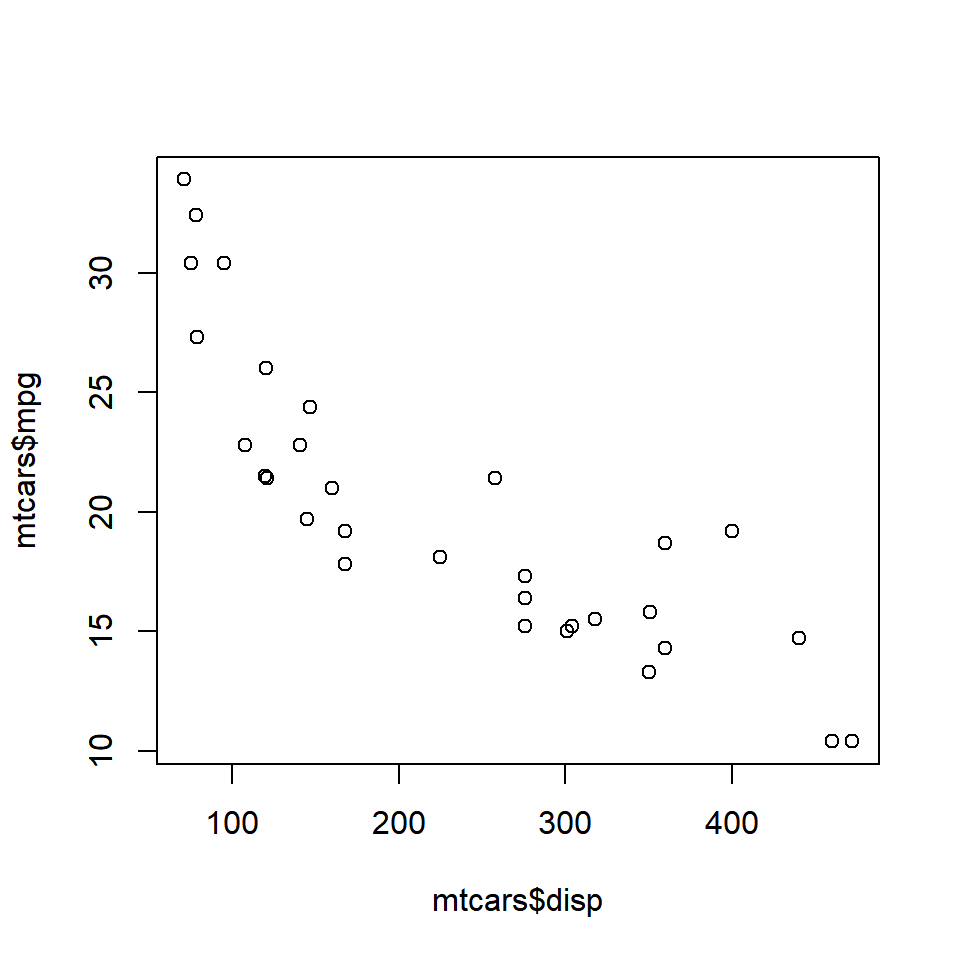## Agenda

• create scatter plot
• modify symbol
• color
• shape
• and shape
• add horizontal & vertical lines
• fit regression line

## Intro

A scatter plot displays the relationship between two continuous variables. In ggplot2, we can build a scatter plot using `geom_point()`. Scatterplots can show you visually

• the strength of the relationship between the variables
• the direction of the relationship between the variables
• and whether outliers exist

## Scatter Plot

``plot(mtcars\$disp, mtcars\$mpg)``## Title & Axis Labels

``````plot(mtcars\$disp, mtcars\$mpg,
main = 'Displacement vs Miles Per Gallon',
xlab = 'Displacement', ylab = 'Miles Per Gallon')``````